# Duffin-Schaeffer conjecture

Letbe a function defined on the positive integers and let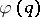be the Euler totient function. The Duffin–Schaeffer conjecture says that for an arbitrary function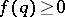(zero values are also allowed for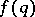), the Diophantine inequality (cf. also Diophantine equations)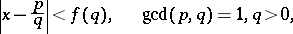(a1)

has infinitely many integer solutionsandfor almost-all real(in the sense of Lebesgue measure) if and only if the series(a2)

diverges. By the Borel–Cantelli lemma, (a1) has only finitely many solutions for almost-allif (a2) converges, and by the Gallagher ergodic theorem, the set of all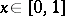for which (a1) has infinitely many integer solutions has measure eitheror.

The Duffin–Schaeffer conjecture is one of the most important unsolved problems in the metric theory of numbers (as of 1998). It was inspired by an effort to replace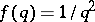by a smaller functionfor which every irrational numbercan be approximated by infinitely many fractions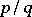such that (a1) holds. This question was answered by A. Hurwitz in 1891, who showed that the best possible function is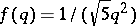. The application of Lebesgue measure to improve thiswas made by A. Khintchine [a7] in 1924. He proved that if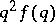is non-increasing and(a3)

diverges, then (a1) has infinitely many integer solutions for almost-all. In 1941, R.J. Duffin and A.C. Schaeffer [a1] improved Khintchine's theorem forsatisfyingfor infinitely manyand some positive constant. They also have given an example of ansuch that (a3) diverges but (a2) converges which naturally leads to the Duffin–Schaeffer conjecture. Up to now (1998), this conjecture remains open. A breakthrough was achieved by P. Erdös [a2], who proved that the conjecture holds, given the additional conditionorfor some. V.G. Sprindzuk comments in [a11] that the answer may depend upon the Riemann hypothesis (cf. also Riemann hypotheses). He also proposes the following-dimensional analogue of the Duffin–Schaeffer conjecture: There are infinitely many integers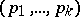andsuch thatand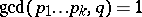for almost-all real numberswhenever the series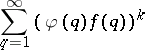(a4)

diverges. A.D. Pollington and R.C. Vaughan [a10] have proved this-dimensional Duffin–Schaeffer conjecture for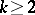. The corresponding result with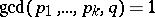instead of the conditionwas given by P.X. Gallagher [a3].

Various authors have studied the problem that the number of solutions of (a1) with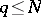is, for almost-all, asymptotically equal to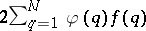.

The problem of restricting both the numeratorsand the denominatorsin (a1) to sets of number-theoretic interest was investigated by G. Harman. In [a6] he considers (a1) where,are both prime numbers. In this case, the Duffin–Schaeffer conjecture has the form: If the sum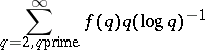(a5)

diverges, then for almost-allthere are infinitely many prime numbers,which satisfy (a1). Harman has proved this conjecture under certain conditions on.

A class of sequences,, of distinct positive integers and a class of functionsis said to satisfy the Duffin–Schaeffer conjecture if the divergence of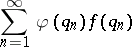(a6)

implies that for almost-allthere exist infinitely manysuch that the Diophantine inequality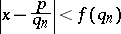has an integer solutionthat is mutually prime with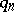(cf. also Mutually-prime numbers). There are tree types of results regarding,satisfying this conjecture (cf. [a5]):

i) any one-to-one sequenceand special(e.g.);

ii) anyand a special(e.g.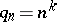,);

iii) special,(e.g.for some). As an interesting consequence of the Erdös result, for almost-allinfinitely many denominators of the continued fraction convergents tolie in the sequenceif and only if (a6) diverges.

Following J. Lesca [a8], one may extend the Duffin–Schaeffer conjecture to the problem of finding sequences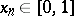such that for every non-increasing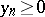, the divergence of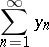(a7)

implies that for almost-allthere exist infinitely manysuch that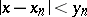. These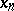are called eutaxic sequences. This problem encompasses all of the above conjectures.

As an illustration, letbe a sequence of reduced fractions with denominators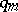,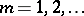, and letforwith a fixed denominator. This gives the classical Duffin–Schaeffer conjecture, since (a6) and (a7) coincide. It is known that the sequence of fractional parts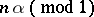is eutaxic if and only if the irrational numberhas bounded partial quotients.

Finally, H. Nakada and G. Wagner [a9] have considered a complex version of the Duffin–Schaeffer conjecture for imaginary quadratic number fields.

The basic general reference books are [a11] and [a4].